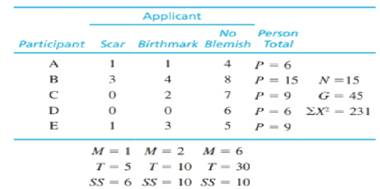Chapter 13, Problem 8PEssentials of Statistics for the B...

8th Edition
Frederick J Gravetter + 1 other
ISBN: 9781133956570

Solutions

Chapter
SectionEssentials of Statistics for the B...

8th Edition
Frederick J Gravetter + 1 other
ISBN: 9781133956570
Textbook Problem

A recent study examined how applicants with a facial blemish such as a scar or birthmark fared in job interviews (Madera & Hebl, 2011). The results indicate that interviewers recalled less information and gave lower ratings to applicants with a blemish. In a similar study, participants conducted computer-simulated interviews with a series of applicants including one with a facial scar and one with a facial birthmark. The following data represent the ratings given to each applicant. a. Use a repeated-measures ANOVA with α = .05 to determine whether there are significant mean differences among the three conditions. b. Compute η2, the percentage of variance accounted for by the mean differences, to measure the size of the treatment effects. c. Write a sentence demonstrating how a research report would present the results of the hypothesis lest and the measure of effect size.a.

To determine
Whether there are significant mean difference among the three conditions using ANOVA at α=0.05.

Explanation

Given info:

The data is provided in the question:

 Participant Applicant Person total N=15G=45∑X2=231 Scan Birthmark No Blemish A 1 1 4 p=6 B 3 4 8 p=15 C 0 2 7 p=9 D 0 0 6 p=6 E 1 3 5 p=9 M=1T=5SS=6 M=2T=10SS=10 M=6T=30SS=10

Calculation:

Step 1:

The null and alternative hypotheses are:

Null hypothesis:

H0:μ1=μ2=μ3,

Where μ1μ2μ3 are the mean ratings given to applicants with scar, birthmark and no blemish, respectively.

Alternate hypothesis:

Ha: At least one treatment mean is different.

Step 2:

First stage:

Compute the degrees of freedom (df) for the between treatment effects, within treatment effects and total and corresponding sum of squares (SS).

Now, it is known that, for a given sample size, the degrees of freedom (df) is:

df=(number of units)1.

Thus,

dftotal=N1=151=14

The number of treatments, k=3. Thus,

dfbetween=k1=31=2

As within treatments degrees of freedom, dfwithin=dftotaldfbetweentreatment, thus,

dfwithin=dftotaldfbetweentreatment=142=12

The total sum of squares is:

SStotal=X2G2N.

The within treatment sum of squares is:

SSwithintreatment=SSinsideeachtreatment.

The within treatment sum of squares is:

SSbetween=T2nG2N.

Thus, here,

SStotal=X2G2N=23145215=96

SSwithintreatment=SSinsideeachtreatment=6+10+10=26

SSbetween=T2nG2N=525+1025+102545215=205135=70

Second stage:

Compute the values of between subject sum of squares and sum of squares due to error

b.

To determine

To find: The value of η2, the percentage of variance accounted for by the mean differences, to measure the size of the treatment effects.

c.

To determine

To write: The results in from of a report.

Still sussing out bartleby?

Check out a sample textbook solution.

See a sample solution

The Solution to Your Study Problems

Bartleby provides explanations to thousands of textbook problems written by our experts, many with advanced degrees!

Get Started

In Exercises 516, evaluate the given quantity. log218

Finite Mathematics and Applied Calculus (MindTap Course List)

Multiply: 25.030.42_

Elementary Technical Mathematics

Evaluate the integral. 01xx2dx

Calculus (MindTap Course List)

Write as an iterated integral, where D is shown at the right.

Study Guide for Stewart's Multivariable Calculus, 8th